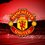# Prove this closed form of $\displaystyle \int_0^1\frac{(\ln(1+x))^2\ln(x)\ln(1-x)}{1-x} \, dx$

$\int_0^1\frac{(\ln(1+x))^2\ln(x)\ln(1-x)}{1-x} \, dx=\dfrac{7}{2}\zeta(3){(\ln 2)^2}-\dfrac{\pi^2}{6}{(\ln 2)^3}-\dfrac{\pi^2}{2}\zeta(3)+{6}\zeta(5)-\dfrac{\pi^4}{48}\ln2$

Prove that the equation above is true.

Notation: $\zeta(\cdot)$ denotes the Riemann Zeta function.

This was found in another mathematics form and it was unanswered there.

This is a part of the set Formidable Series and Integrals.5 years, 5 months ago

This discussion board is a place to discuss our Daily Challenges and the math and science related to those challenges. Explanations are more than just a solution — they should explain the steps and thinking strategies that you used to obtain the solution. Comments should further the discussion of math and science.

When posting on Brilliant:

• Use the emojis to react to an explanation, whether you're congratulating a job well done , or just really confused .
• Ask specific questions about the challenge or the steps in somebody's explanation. Well-posed questions can add a lot to the discussion, but posting "I don't understand!" doesn't help anyone.
• Try to contribute something new to the discussion, whether it is an extension, generalization or other idea related to the challenge.

MarkdownAppears as
*italics* or _italics_ italics
**bold** or __bold__ bold
- bulleted- list
• bulleted
• list
1. numbered2. list
1. numbered
2. list
Note: you must add a full line of space before and after lists for them to show up correctly
paragraph 1paragraph 2

paragraph 1

paragraph 2

[example link](https://brilliant.org)example link
> This is a quote
This is a quote
    # I indented these lines
# 4 spaces, and now they show
# up as a code block.

print "hello world"
# I indented these lines
# 4 spaces, and now they show
# up as a code block.

print "hello world"
MathAppears as
Remember to wrap math in $$ ... $$ or $ ... $ to ensure proper formatting.
2 \times 3 $2 \times 3$
2^{34} $2^{34}$
a_{i-1} $a_{i-1}$
\frac{2}{3} $\frac{2}{3}$
\sqrt{2} $\sqrt{2}$
\sum_{i=1}^3 $\sum_{i=1}^3$
\sin \theta $\sin \theta$
\boxed{123} $\boxed{123}$

Sort by:

Use this expansion,

$\large \ln ^2 (1+x) = \sum_{r=0}^{\infty} \dfrac{H_{r} (-1)^r x^{r+1}}{r+1}$

Then it will be left to evaluate the integral

$\large \int_{0}^{1} x^{r+1} \dfrac{\ln(x) \ln(1-x)}{1-x} dx$

This is derivative of beta function.

- 5 years, 5 months ago

How will you evaluate the resulting summation?

- 5 years, 5 months ago

wow nice one! Do you know how to prove it?

- 5 years, 5 months ago

@Pi Han Goh add it to you set

- 5 years, 5 months ago

- 5 years, 5 months ago

Thanks. Do try it.

- 5 years, 5 months ago

Now @Ishan Singh can solve this.. Integral can be written as:

$\displaystyle \sum_{r,s,t\geq 0} \frac{H_r(-1)^r}{(r+1)(t+1)(r+s+t+3)^2}$

- 5 years, 5 months ago

@Mark Hennings could you help us out?

- 5 years, 5 months ago

Hint : Convert into derivative of Beta function.

- 5 years, 5 months ago

Yup I did that but at some point, I have to take natural logarithm of (-1).

Which is imaginary, but the closed form ain't contain any imaginary term.

- 5 years, 5 months ago

No. Take limit. For example this

- 5 years, 5 months ago

You have to do something with the $\ln^2 (1+x)$ term and afterwards it gets converted into the limit. Another method is to use generating function of Harmonic numbers.

- 5 years, 5 months ago

Kk I'll give another shot at Feynman's way.

- 5 years, 5 months ago

I got imaginary term while using that Harmonic relation.

You are referring this $\sum_{n=1}^{\infty} H_{n} x^{n} = \dfrac{-ln(1-x)}{1-x}$ no?

- 5 years, 5 months ago

Yes. I'm referring to that generating function.

- 5 years, 5 months ago

can u clearly explain how u applied the limit there?

- 5 years, 5 months ago

That will take $2 -3$ pages. I may post it when I'm free.

- 5 years, 5 months ago